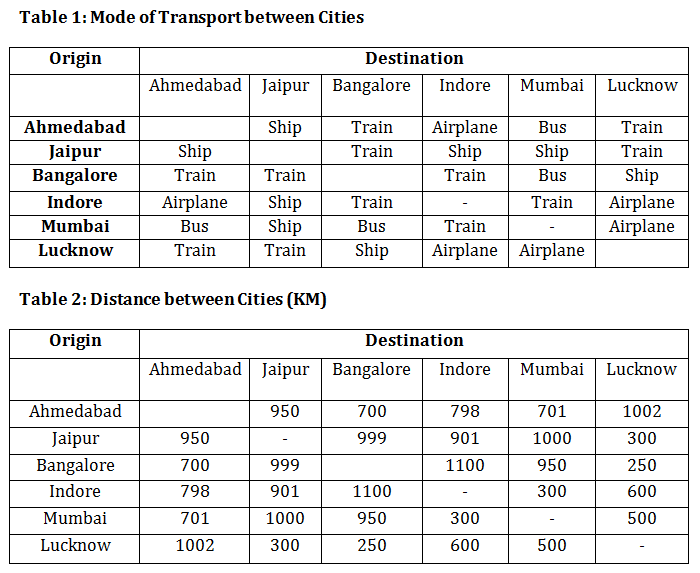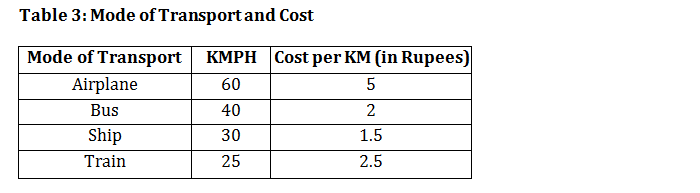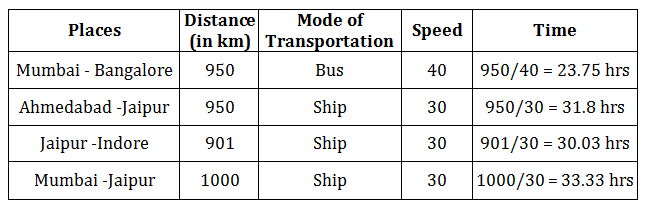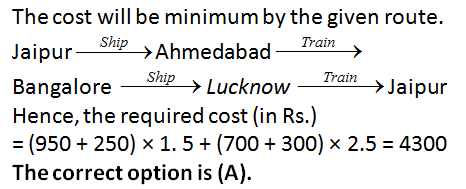### SSC reasoning ability questions with answers: SSC reasoning ability questions with answers to master logical concepts for exams

SSC reasoning ability questions with answers are a great source for efficient exam preparation. The question sets cover the entire syllabus that is important for the SSC Exam and other competitive exams. The more you practice, the more concepts you will cover and be confident in solving similar type of sums in the exam. Practice SSC reasoning ability questions with answers as there are questions ranging from easy to difficult to help you be efficient in all categories of problems. You need to display good speed in easy sums so that you can invest the saved time in solving difficult problems and on the other hand you need to display strong concept understanding and good thinking pattern while solving difficult problems. You can achieve this through SSC reasoning ability questions with answers.

Directions for the question set:
Ahmedabad, Jaipur, Bangalore, Indore, Mumbai and Lucknow are 6 major Indian cities. For some reason people use only a certain mode of transport between a pair of cities. The modes of transport are provided in Table 1, while in Table 2 the distances between different pairs of cities are given. Table 3 provides the speed of the mode of transport and the cost associated with each of them.Question 1: Mr. Ranjith lives in Mumbai and wants to travel to Indore. However, the train services are on halt due to laying of track for bullet trains across the country. In this scenario, which of the following is the least cost route to reach Indore?
A. Mumbai – Ahmedabad – Indore
B. Mumbai – Jaipur – Indore
C. Mumbai – Bangalore – Indore
D. Mumbai – Lucknow- Indore

Question 2: For which of the following options, travel time is the least?
A. Mumbai – Bangalore
C. Jaipur-Indore
D. Mumbai-Jaipur

Question 3: What is the least cost way to reach to Lucknow from Indore?
A. Take a flight from Indore to Lucknow
B. Take a ship from Indore to Jaipur and then take a train to Lucknow
C. Take a train from Indore to Bangalore and then take a ship to Lucknow
D. Take a train from Indore to Mumbai and then take a flight to Lucknow

Question 4: A school in Jaipur is planning for an excursion tour for its students. They want to show them Bangalore, Lucknow, and Ahmedabad, not necessarily in the same order. What is the minimum travel cost (in Rs.) the school should charge from each of the students for the entire tour?
A. Rs. 4300
B. Rs. 5000
C. Rs. 7500
D. Rs. 6800

Question 5: Which of the following cities can be reached from Ahmedabad in least time?
A. Jaipur
B. Bangalore
C. Mumbai
D. Lucknow

### Answers and Explanations: Click the down arrow to expand

Answers 1: (B) We will have to consider each option and then we can reach a conclusion about the least cost path. Costs of each route given in the options is as follows:

(i) Mumbai – Ahmedabad (Bus) and Ahmedabad – Indore (Airplane) Total fare = 701 × 2 + 798 × 5 = 1402 + 3990 = 5392

(ii) Mumbai – Jaipur (Ship) and Jaipur – Indore (ship) Total fare = 1000 × 1.5 + 901 × 1.5 = 1500 + 1351.5 = 2851.5

(iii) Mumbai – Bangalore (Bus) and Bangalore – Indore (Train) Total = 950 × 2 +1100 × 2.5 = 1900 + 2750 = 4650

(iv) Mumbai – Lucknow (Airplane) and Lucknow – Indore (Airplane) Total fare = 500 × 5 + 600 × 5 = 5500

From the above, Mumbai – Jaipur – Indore is the best deal. The correct option is (B).

Answers 2: (A) Time taken in each of the given options case is shown below:The correct option is (A).

Answers 3: (B) The costs for each of the given route is shown below. Indore – Lucknow = 600 × 5 = Rs. 3000. Indore – Jaipur – Lucknow = 901 × 1.5 + 300 × 2.5 = Rs. 2101.5. Indore – Bangalore – Lucknow = 1100 × 2.5 + 250 × 1.5 = Rs. 3125. Indore – Mumbai – Lucknow = 300 × 2.5 + 500 × 5 = Rs. 3250. The correct option is (B).